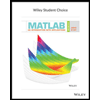Question

Automobile repair costs continue to rise with the average cost now at \$367 per repair.† Assume that the cost for an automobile repair is normally distributed with a standard deviation of \$88. Answer the following questions about the cost of automobile repairs.

(a)What is the probability that the cost will be more than \$460? (Round your answer to four decimal places.)

(b)What is the probability that the cost will be less than \$240? (Round your answer to four decimal places.)

(c)What is the probability that the cost will be between \$240 and \$460? (Round your answer to four decimal places.)

(d)If the cost for your car repair is in the lower 5% of automobile repair charges, what is your maximum possible cost in dollars? (Round your answer to the nearest cent.)
Expert Solution

### Want to see the full answer?

Check out a sample Q&A hereStudents who’ve seen this question also like:MATLAB: An Introduction with Applications
6th Edition
ISBN: 9781119256830
Author: Amos Gilat
Publisher: John Wiley & Sons Inc